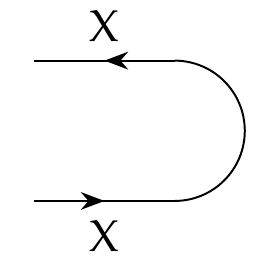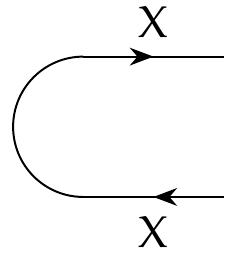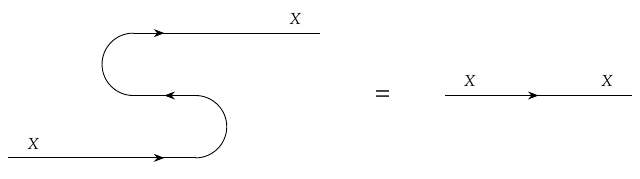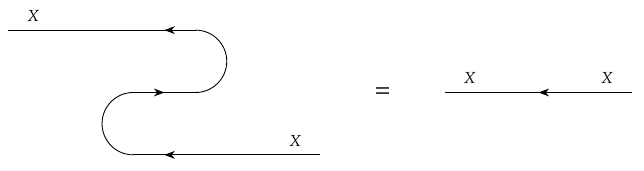Okay! I think I've got this lecture fixed, with consistent conventions.

* The cup:

$\cup_X \colon X^{\text{op}} \times X \nrightarrow \textbf{1}$* The cap:

$\cap_X \colon \textbf{1} \nrightarrow X \times X^{\text{op}}$These conventions are different than the book's, because in the book's discussion of compact closed categories they use a 'unit' which corresponds to a cup \$$\cup_X \colon X \times X^{\text{op}} \nrightarrow \textbf{1} \$$ and a 'counit' which corresponds to a cap \$$\cap_X \colon \textbf{1} \nrightarrow X^{\text{op}} \times X \$$. These are equally consistent convention, but much less commonly used in the category theory literature, for good reasons I won't explain now, so I think it's better for us to use the more standard ones.

Either way, the snake equations are then the two equations one can write down using \$$\cap_X\$$ and \$$\cup_X\$$. For me they are:

* The first snake equation:

$(1\_X \times \cup\_X) (\cap\_X \times 1_X) = 1\_X .$* The second snake equation:

$(\cup_X \times 1\_{X^{\text{op}}}) (1\_{X^{\text{op}}} \times \cap_X) = 1_{X^{\text{op}}} .$